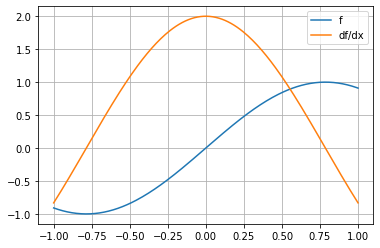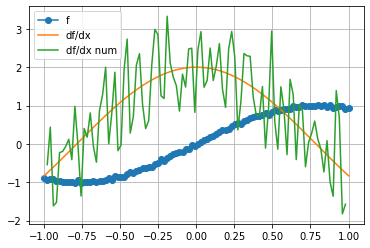# Finite differences vs Noise¶

In :
import numpy as np
import numpy.linalg as la
import matplotlib.pyplot as pt

In :
def f(x):
return np.sin(2*x)
def df(x):
return 2*np.cos(2*x)


Here's a pretty simple function and its derivative:

In :
plot_x = np.linspace(-1, 1, 200)

pt.plot(plot_x, f(plot_x), label="f")
pt.plot(plot_x, df(plot_x), label="df/dx")
pt.grid()
pt.legend()

Out:
<matplotlib.legend.Legend at 0x11fd9c550>Now what happens to our numerical differentiation if our function values have a slight amount of error?

In :
# set up grid
n = 100
x = np.linspace(-1, 1, n)
h = x - x
x_df_result = x[1:-1] # chop off first, last point

# evaluate f, perturb data, finite differences of f
f_x = f(x)

f_x += 0.025*np.random.randn(n)

df_num_x = (f_x[2:] - f_x[:-2])/(2*h)

# plot
pt.plot(x, f_x, "o-", label="f")
pt.plot(plot_x, df(plot_x), label="df/dx")
pt.plot(x_df_result, df_num_x, label="df/dx num")
pt.grid()
pt.legend(loc="best")

Out:
<matplotlib.legend.Legend at 0x11ff9e610>• Now what happens if you set n = 100 instead of n = 10?